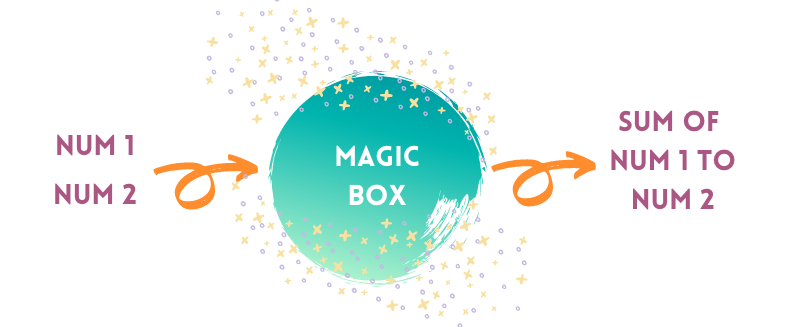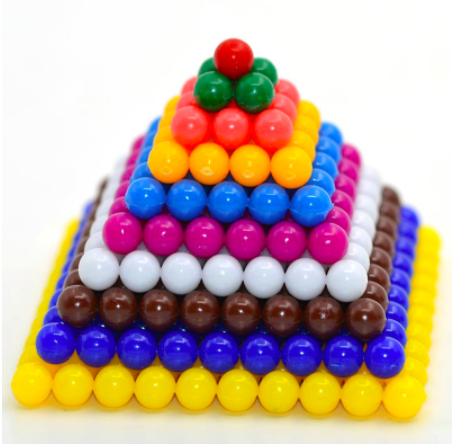# Methods

## What is a Method?

So far, we’ve learned about how to store data in variables, print data and sentences, and make decisions with if-statement, for-loop, and while-loop.

From the last exercise, we know the following block of code calculates the sum from 1 to 100:

int total = 0;
int num = 1;
while(num <= 100){
total = total + num;
num = num + 1;
}
System.out.println("Sum: " + total);


But what if we want to calculate the sum from 1 to 77 instead? How do we do it without writing the whole blocks of code again?

We want to create a ✨ magic box ✨ in code that does the calculation for us no matter which 2 numbers we want to create a sum for!In Java, a method is like the magic box that performs a specific task by running a block of code that can use user inputs.

There are 2 parts in a method: header and body:

## Count the Pyramid!🔺(photo credit: aliexpress.com/item/32306945847.html)

The above is an image of square-number pyramid where each level is a perfect square of the number of current level counting from the top.

That is the top level has 1 * 1 bead, the 2nd level has 2 * 2 beads.

Let’s write a method that takes in the number of total levels and output the total number of beads in the pyramid!

Once you solved the challenge, you will see the following message:

Congratulations! Challenge Solved!